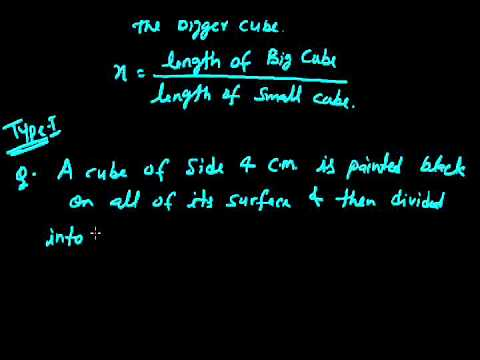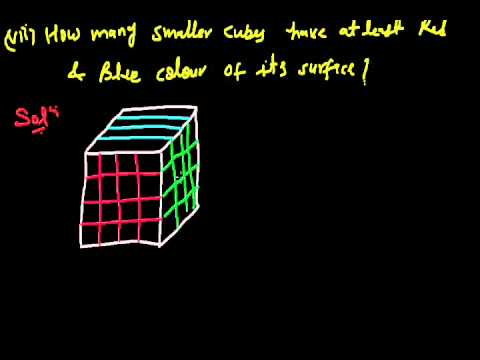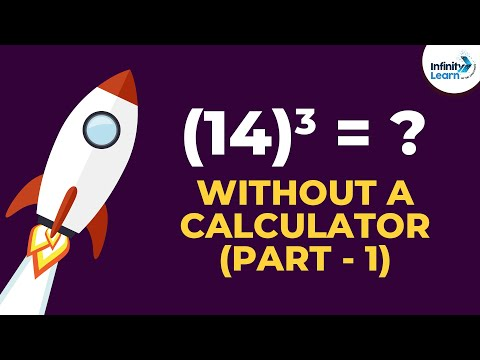Courses

# Test: Cubes- 1

## 5 Questions MCQ Test UPSC Prelims Paper 2 CSAT - Quant, Verbal & Decision Making | Test: Cubes- 1

Description
This mock test of Test: Cubes- 1 for UPSC helps you for every UPSC entrance exam. This contains 5 Multiple Choice Questions for UPSC Test: Cubes- 1 (mcq) to study with solutions a complete question bank. The solved questions answers in this Test: Cubes- 1 quiz give you a good mix of easy questions and tough questions. UPSC students definitely take this Test: Cubes- 1 exercise for a better result in the exam. You can find other Test: Cubes- 1 extra questions, long questions & short questions for UPSC on EduRev as well by searching above.
QUESTION: 1

### Q. If volume of cube is 4913cm3 then length of side of cube is

Solution:

Let side be 'a'
volume of a cube=a3
a3 = 4913
a=  ∛4913
a = 17cm

QUESTION: 2

Solution:

343 = 7 ∗ 7 ∗ 7
343 = (7)³
=> ³√343 = 7

QUESTION: 3

### If length of one side of cube is 20cm then volume of cube must be

Solution:

Volume of cube = a3

Given that side of a cube is 20cm

Then volume will be (20cm)3

Volume = 8000 cm3

QUESTION: 4

93 (cube of 9) is equal to

Solution:

9×9×9=729

QUESTION: 5

Cubic root of 729 is

Solution:

An integer is divisible by 9 if and only if the sum of its digits is divisible by 9.

The sum of the digits of 729 is 7 + 2 + 9 = 18 and 18 is divisible by 9 so 729 is divisible by 9.

Hence, the correct answer is Option D

Track your progress, build streaks, highlight & save important lessons and more!

### Similar Content### Related tests